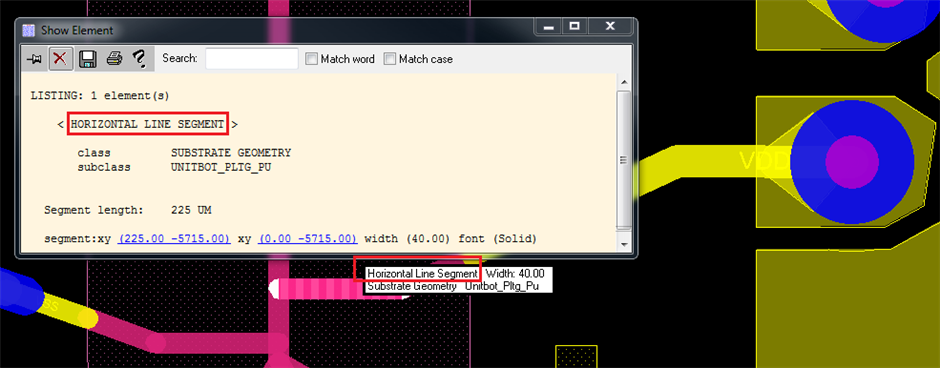How to check the <NAME> of a line?

How do I check the < NAME > name of line?  Is there a line_refdes like component_refdes? Something like:(define PlatingTrace
(select (mPlatingTrace) line
(ZZZZZZ_refdes? mPlatingTrace "HORIZONTAL LINE SEGMENT"))

(layer? mPlatingTrace "SUBSTRATE GEOMETRY/UNITBOT_PLTG_PU")
)

THANKS, RIAN

Parents
• Hi Rian,

that is not a name, but rather a description. A segment can be described as horizontal, vertical, odd-angle or arc. In Ravel, you can find if a segment is horizontal by seeing if it is parallel to a horizontal unit vector:

(axis_angle segment (CREATE_VECTOR 1 0)) < 1.0

In my previous post I forgot to mention that CREATE_VECTOR is a macro in standard/geometry in Ravel Standard Library. To create a vector without using the standard library, you would do:

(connecting_line (create_point 0 0) (create_point 1 0))

Regards,

Björn

• Hi Rian,

that is not a name, but rather a description. A segment can be described as horizontal, vertical, odd-angle or arc. In Ravel, you can find if a segment is horizontal by seeing if it is parallel to a horizontal unit vector:

(axis_angle segment (CREATE_VECTOR 1 0)) < 1.0

In my previous post I forgot to mention that CREATE_VECTOR is a macro in standard/geometry in Ravel Standard Library. To create a vector without using the standard library, you would do:

(connecting_line (create_point 0 0) (create_point 1 0))

Regards,

Björn

Children
No Data
Related